# Counterfactual knowability revisited

## Abstract

Anti-realism is plagued by Fitch’s paradox: the remarkable result that if one accepts that all truths are knowable, minimal assumptions about the nature of knowledge entail that every truth is known. Dorothy Edgington suggests to address this problem by understanding p is knowable to be a counterfactual claim, but her proposal must contend with a forceful objection by Timothy Williamson. I revisit Edgington’s basic idea and find that Williamson’s objection is obviated by a refined understanding of counterfactual knowability that is grounded in possible courses of inquiry. I arrive at a precise definition of knowability that is not just a technical avoidance of paradox, but is metaphysically sound and does justice to the anti-realist idea.

## Introduction

Anti-realists endorse the thesis that all truths are knowable (Dummett 1991; Tennant 1997). The celebrated result known as Fitch’s paradox appears to show that the anti-realist thesis is incompatible with the nigh-unassailable claim that there is an unknown truth. Many solutions to the paradox have been proposed (Tennant 1997; Beall 2000; Fara 2010; Fischer 2013; Artemov and Protopopescu 2013, to name just a few), but ‘there continues to be no consensus on whether and where’ things go wrong for the anti-realist (Brogaard and Salerno 2013). There is equally little consensus on just what kind of modality is the able in knowable.

In this paper, I revisit—and attempt to vindicate—the suggestion of Dorothy Edgington (1985) that knowability should be understood to denote counterfactual knowledge of actual truth. This proposal has several attractive features. It is a factive account of knowability, i.e. all knowable propositions are actually true (as they should; cf. Fuhrmann 2014),Footnote 1 and it unrestrictedly makes all actual truths knowable. This allows the anti-realist to identify truth and knowability (as she should; cf. Kvanvig 1995). Moreover, characterising knowability as counterfactual knowledge of actuality requires no more than the usual vocabulary of counterfactual semantics, whereas some other proposals must introduce new modalities or new takes on metaphysical possibility (e.g. Rückert 2004; Tennant 2009; Fara 2010; Fischer 2013; Fuhrmann 2014).Footnote 2

However, Edgington’s proposal must contend with a powerful objection by Timothy Williamson (1987). He argues that there cannot be any non-trivial counterfactual knowledge of actuality and I demonstrate that a certain strengthening of this objection remains forceful against Edgington’s (2010) more recent response. However, I then continue to argue that if counterfactual knowledge is defined with respect to those possibilities that arise from the counterfactual pursuit of some knowledge-imparting process, the objection is met. Appealing to knowledge-imparting processes should come as an entirely natural step for the anti-realist, who generally appeals to (idealised) processes of verification (Kvanvig 1995; Tennant 1997; Fischer 2013).

I consider my task to be one of explication. Fitch’s paradox shows that the anti-realist’s use of knowable is in need of clarification, and further objections raised against the anti-realist might require further clarification. In what follows, I go through some iterations of this process to ultimately arrive at a formulation of the anti-realist’s thesis that appears to resist attempts to trivialise it. Technical vindications of Edgington’s proposal have been developed (Rabinowicz and Segerberg 1994; Rückert 2004), but according to Williamson (2009) these solutions fail to ‘fully answer the philosophical objections’ (fn. 10). He does not elaborate how exactly they fail to meet these objections. In any case, the proposal developed here is not merely a formal avoidance of paradox, but a metaphysically and philosophically appreciable explication of what is meant by knowable that allows one to coherently claim that truth is what is knowable.

## On the meaning of knowable

### Counterfactual knowability

As persuasively argued by Dummett (1991) and Tennant (1997), among others, there seem to be good reasons (linguistic, metaphysical, epistemological) to suppose that truth is just what is knowable. I call this the anti-realist thesis; defenders of it are known as anti-realists. (It is worth pointing out that Edgington, whose proposal I defend and elaborate, does not have the goal to advance a thesis about truth (Edgington 2010, p. 51), although her ideas are useful to those who do.) Anti-realists must contend with the Fitch objection to their thesis. It goes as follows.

We may suppose the following uncontroversial assumptions about epistemic logic. Knowledge is factive ($$Kp \rightarrow p$$); knowledge distributes conjunctively ($$K(p \wedge q) \rightarrow (Kp \wedge Kq)$$); and if something is a logical contradiction, it is impossible ($$p\vdash \bot$$ entails $$\vdash \lnot \Diamond p$$). Now consider the proposition p and p is not known, i.e. $$p\wedge \lnot Kp$$, and note that $$K(p \wedge \lnot Kp)$$ entails that $$Kp \wedge K\lnot Kp$$ by distribution. The latter’s right conjunct entails $$\lnot Kp$$ by factiveness. Hence we have $$Kp \wedge \lnot Kp$$, i.e. a contradiction. Thus, $$\vdash \lnot \Diamond K(p \wedge \lnot Kp)$$, i.e. it is impossible to know $$p \wedge \lnot Kp$$. Hence, many are inclined to conclude, there is an unknowable truth—namely p and p is not known for some true but unknown p—and so anti-realism fails.

The argument is valid as it stands, but yet the anti-realist should protest. Of course our knowledge can change. So if there is an unknown truth p, we should say that it is unknown in our present epistemic state s. No similar triviality result follows from the claim that at a state that is not our present state it is known that p and p is not known in s. However, this solution needs to clarify what is meant by present.

It can easily be seen that present cannot simply be a time index (Kvanvig 1995). For if we were to write $$K^t p$$ for “p is known at time t”, we could consider the sentence p and p is never known, formalised as $$p \wedge \forall t(\lnot K^t p)$$. Then, analogously to the standard Fitch objection, one can show that it is impossible to know at some time that p and p is never known. So, if we insist that every truth is knowable for a tensed sense of knowable, we have to accept that $$p \wedge \forall t(\lnot K^t p)$$ is no truth. Worse still, if we take every truth is knowable to be a thesis about a metaphysical necessity (as we should, if it is to be understood as a thesis about the nature of truth), we need to accept that it is impossible that $$p \wedge \forall t(\lnot K^t p)$$ is true. Hence, it follows that necessarily it is the case that $$p \rightarrow \exists t(K^t p)$$.

However, it is certainly possible that some truths will never be known. For instance, all sentient life may cease to exist tomorrow—long before we have discovered all truths. So whatever sense can be made of knowable when faced with the Fitch objection, it cannot be found in differentiating what we know now from what we will know.

As a first attempt to solve this puzzle, one can appeal to non-actual times. When we say that actually a truth won’t ever be known, we are quantifying over the actual times of the actual world. So if we want to claim that it is knowable that p and p won’t be known at any actual time t, then the time index $$t'$$ at which this proposition is known is not an actual time, i.e. not among the t.

There are some formal difficulties with this, pointed out by Kvanvig (1995). If we want to quantify over times, we run into the challenges of quantifying into modal position. But for present purposes we can sidestep that issue by using temporal modals. That is, if F is a modal ‘at some time’, then the claim p won’t ever be known is $$\lnot F Kp$$. Then, to claim that at some time it is known that actually $$\lnot FKp$$ is to claim that $$\Diamond FK(A\lnot FKp)$$ (for A being the in the actual world operator). The anti-realist may claim that the outer F makes different times accessible than the inner F (since A forces the inner F to be interpreted with respect to the actual world). This is the case in a standard modal semantics, and there is no formal or metaphysical problem with this.

However, this means that the anti-realist who thusly insists on the knowability of p and p is never known is making a counterfactual claim: p is knowable if p is known at some possibly non-actual time. Hence knowable includes a metaphysical possibility operator. The anti-realist may appeal to examples like the following to give plausibility to the counterfactual move.

(Oats).:

Say that I had muesli for breakfast. There is some number N such that N is exactly the number of oats in my bowl. Hence, $$p=$$Julian ate N oats this morning is a truth. But I didn’t count the oats and ate all the evidence, so this won’t ever be known in this world.Footnote 3

Edgington (2010) is discontent, and quite rightly so, with any notion of knowability on which such “very mundane facts” such as Julian ate N oats this morning “should be in principle unknowable” (p. 51). There is a good sense in which p is knowable in (Oats): one could have counted, hence could have known, for a metaphysical reading of could.

However, it would be a mistake to therefore write the anti-realist thesis as $$p \leftrightarrow \Diamond Kp$$ for $$\Diamond$$ being the usual operator for metaphysical possibility. It is metaphysically possible that grass is purple. Hence it is also metaphysically possible that someone knows that $$p=$$grass is purple. Hence there is a world in which Kp, hence it is the case that $$\Diamond Kp$$, but certainly grass is purple is not true in the actual world, hence it should not count as knowable (Tennant 2009; Fara 2010; Fuhrmann 2014).

Dorothy Edgington (1985) therefore puts the anti-realist thesis as every actual truth is knowable to be an actual truth. Writing A for ‘in the actual world’, K for ‘it is known at some time’ (incorporating tense) and $$\Diamond$$ for metaphysical possibility, she can state the formal form of the anti-realist thesis as (AK).

(AK) $$Ap \rightarrow \Diamond KAp$$.

This avoids the Fitch objection because KA is not a factive operator in non-actual worlds. If it is actually true that p and p won’t be known, (AK) entails that $$\Diamond KA(p and p won't be known )$$, which is consistent because in non-actual worlds, the complex operator KA is not factive. Hence KAp and $$KA(p won't be known )$$ are consistent in non-actual worlds. Moreover, $$\Diamond AK$$ is a factive notion of knowability. In worlds where, say, grass is purple, it cannot be known that actually grass is purple, since grass is actually green.

But is (AK) philosophically sound? According to the (AK) conception of knowability, a proposition p being knowable means that in some non-actual world someone has knowledge about the actual world. To seriously defend this, the anti-realist must seemingly endorse a strong principle of transworld awareness. What is more, she appears to stipulate that knowers in non-actual worlds are able to determine which world is actual. But if they are, it seems their knowledge of the actual world would be trivial. This can be seen as follows (Rabinowicz and Segerberg 1994; Artemov and Protopopescu 2013).

Generally, it holds for the actuality operator A and any proposition p that $$\Box Ap \leftrightarrow Ap$$. Then, a derivation of triviality from (AK) follows model-theoretically, assuming standard semantics for K (i.e. that Kp is true if at all epistemically accessible worlds, p is true). If p is any actual truth, then Ap is necessary. That is, if p is an actual truth, Ap is true at all worlds v—including at those that are epistemically accessible from the actual world. Hence, KAp is actually true. Thus $$Ap \rightarrow KAp$$ for any p. Call this the simple triviality argument.

Clearly, the anti-realist must further clarify her thesis to make it plausible that inhabitants of non-actual worlds can have non-trivial knowledge of actuality. Edgington (1985) already makes the useful suggestion that the operator A is not centred on the actual world, but on the actual situation. The actual situation consists of the actual facts relevant to the current inquiry, but does not fully specify the actual world (e.g. if the relevant proposition is about which face a coin shows, there are only two relevant situations: heads and tails).

Then, non-actual knowers can have knowledge of actuality merely by being acquainted with a specifying description of the actual situation. One may therefore explicate (AK) to the statement ($$\alpha$$K).

The plausibility of ($$\alpha$$K) stands and falls with how much information is contained in $$\alpha$$. Obviously, we cannot call a p knowable whenever there is some specification $$\alpha$$ of the actual situation for which it holds that $$\Diamond K(\alpha \rightarrow p)$$. This would be a trivial notion of knowability, since we could just include the fact that p in $$\alpha$$. If p is part of $$\alpha$$, then $$K(\alpha \rightarrow p)$$ is knowledge of a triviality, not of actuality (Williamson 1987; Fara 2010). By the very same argument, the anti-realist must reject that $$\alpha$$ logically entails p. Possible knowledge of a logical fact also does not entail anything about the actual world.

Hence, the anti-realist must answer a tough question: what is it for an $$\alpha$$ to specify the actual situation in the sense of ($$\alpha$$K)? More specifically, if $$\alpha$$ does not entail p, how can someone in a non-actual world know that in the actual situation (that she only knows by being acquainted with $$\alpha$$) it is the case that p?

In the coin flip case, if the actual situation is tails, the relevant $$\alpha$$ must specify some facts that allow one to distinguish tails from heads, without entailing tails outright. The question is, what are these facts? To untangle this, it helps to consider an example due to Edgington (1985).

(Comet).:

Suppose there is a scientific experiment that can be performed exactly once, and failure to perform it results in that some knowledge remains unknown forever. To give a specific case, suppose there is a comet that is disintegrating, and we have precisely one shot at landing a probe on it to analyse its composition before it is gone. There is a chance of critical failure (say, the probe breaks on landing), which results in us never being able to analyse the comet (there is no time to send another probe before it disintegrates). Then, let p denote the proposition describing the comets composition, $$s_K$$ be the situation that is specified by the experiment goes as planned and $$s_{\lnot K}$$ be the situation specified by $$\textit{the experiment fails}$$. Suppose moreover that $$s_{\lnot K}$$ specifies the actual situation, i.e. that we actually don’t know p and won’t ever know p. Then $$A(p \wedge \lnot K p)$$ and $$\Diamond K(s_{\lnot K}\rightarrow (p \wedge \lnot Kp))$$. The latter is because it is known in the situation described by $$s_K$$ that if the situation were $$s_{\lnot K}$$, it would (still) be the case that p, but p would never be known.

So, in the case of (Comet) the appropriate specification of actuality is $$\alpha =s_{\lnot K}=$$the experiment fails. Note that the knowledge of actuality in (Comet) is properly paraphrased as a counterfactual conditional evaluated in a non-actual situation. Thus, we should explicate ($$\alpha$$K) further to ($$\alpha$$K$$'$$), using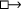to express a counterfactual conditional.

That is, p is knowable if there is a possible situation s where it is known that if things were as in the specification $$\alpha$$ of the actual situation, then p. With respect to s, this conditional is counterfactual. We have seen in (Comet) an example for an appropriate $$\alpha$$. But to defend ($$\alpha$$K’), the anti-realist should give general criteria to select an appropriate $$\alpha$$.

Williamson (1987) argues that this cannot be done, since however one specifies $$\alpha$$, if in the situation specified by $$\alpha$$, p is true, then it is necessary. Hence, knowing that in the situation specified by $$\alpha$$, p holds “requires no more than knowledge of a trivial logical truth” (p. 259). For the argument, suppose a standard account of counterfactual conditionals adapted to situations, i.e.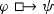is true at a situation s if at the situation $$s'$$ that contains $$\varphi$$ and is is closest to s with that property, $$\psi$$ holds.Footnote 4

Now, the defender of ($$\alpha$$K$$'$$) claims that in a situation s, knowledge that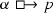constitutes knowledge of the actual situation. For this to be true, $$\alpha$$ needs to be specified such that for any proposition r in the actual situation, the $$\alpha$$-situation closest to s must also contain r, since otherwise $$\alpha$$ would fail to specify the actual situation. Ex hypothesi, the actual situation contains p. Thus the $$\alpha$$-situation closest to s is also the $$(\alpha \wedge p)$$-situation closest to s. This goes for any s and sois necessarily equivalent to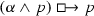. Thus,is necessarily equivalent to a logical truth, sois itself necessary. So, as in the simple triviality argument, it is necessarily the case thatis true in all epistemically accessible worlds; so knowingis trivial. The move from (AK) to ($$\alpha$$K$$'$$) does not seem to have improved things. By a very similar argument, Fara (2010) also arrives at this conclusion.

Before I turn to my own solution to this quandary, it is worth considering Edgington’s (2010) own rebuttal. She responds that it is not prima facie impossible to have non-trivial knowledge of actuality in a non-actual situations, since to her the “received wisdom about the causal and spatio-temporal isolation of counterfactual situations is overdone” (p. 48). She argues that it is quite plausible that some situations share a causal history with the actual situation up to some “point of departure” (p. 51) and, she continues, that someone in a counterfactual situation can specify the actual situation by referring to this shared history and the point of departure. An example where this makes sense is (Comet): someone in the non-actual situation that is specified by the experiment succeeded can consider the experiment as a point of departure, and specify actuality by referencing the experiment and its possible outcomes: “We will think, about the other possibility, that if the experiment had gone wrong, p would never have been known.” (Edgington 2010, p. 48).

That is, Edgington proposes to meet Williamson’s challenge by specifying non-actual situations by appealing to a branching event, e.g. by saying in the other outcome (of some event) (p. 42). But this is not yet precise enough. If the event has more than two possible outcomes, is is unclear how to refer to the actual situation: if I roll a die, there are six possible situations; if I roll two dice, 36—how do I pick out the actual situation, if there is no unique other? (This concern is also raised by Fara 2010). Edgington (2010) contends that one can directly refer to some situations by referring to another outcome of a branching event. But if there are multiple other outcomes, one must somehow distinguish the actual outcome. Supposedly, one distinguishes it by a description $$\alpha$$ (say, the outcome in which...).

A variant of Williamson’s argument shows that even when appealing to shared histories, this $$\alpha$$ must be such that knowingis trivial. The present suggestion is that one can know about other situations by knowing that they share a causal history, where their history branches off, and referring to the branching point. Again, $$\alpha$$ must be specific enough to distinguish the unique actual situation. So for all r in the actual situation, in all situations sharing this history and branching point,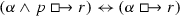, lest knowledge of $$\alpha$$ fail to be knowledge of actuality. Therefore, while this biconditional is not outright necessary (it may fail in causally isolated situations), it is a necessary consequence of sharing the history and branching point. But since we suppose that in these situations it is known that they share a history and branching point, all from them epistemically accessible worlds share this history too, and hence satisfy the biconditional. So knowing thatagain requires no more than knowing a triviality.

Thus, Williamson’s challenge remains forceful, even given Edgington’s appeal to shared histories. I argue that the objection can nevertheless be met. In what follows, I propose a suitable clarification of ($$\alpha$$K$$'$$) that follows many of Edgington’s intuitions, but without pretending that this is what she might mean or should mean. My account replaces $$\Diamond$$ in ($$\alpha$$K$$'$$) with another counterfactual conditional. This allows me to give a general definition of what the specification $$\alpha$$ is and to define in which particular worlds knowledge ofcounts as knowledge of actuality. Williamson’s objection is then avoided by denying that knowledge of $$\alpha$$ must necessarily be knowledge of actuality.

### The counterfactual pursuit of inquiries

For the sake of clarity, I return to talk of possible worlds instead of situations, but my proposal could be executed in a situation semantics as well. Now, my discussion of the knowability principle has been so far silent on the means by which we gain knowledge. As the examples (Oats) and (Comet) suggest, the anti-realist should not be interested in non-actual worlds (or situations) where it just so happens that someone knows. Rather, the relevant worlds are those that differ from the actual world in that someone has done something to get that knowledge (experimenting, counting, measuring, ...).

Thus, instead of appealing to the unclear notion of a shared history, I suggest that the worlds where knowledge of $$\alpha$$ constitutes knowledge of the actual world are those worlds that differ from the actual world in the successful performance of some action that brings about the requisite knowledge. In those worlds, someone might know that (even) if I hadn’t done such-and-such, it would (still) be true that this-and-that. In (Oats), in a counterfactual situation where I counted, I know that even if I hadn’t counted, I would still have eaten N oats. Since I actually did not count, knowledge of this counterfactual constitutes knowledge of actuality (and I argue that this is non-trivial knowledge). This plan can be spelled out formally in the language of counterfactual conditionals.

For the purposes of a formal statement, we can abstractly consider all possible courses of inquiry. Write $$\textit{Inq}$$ for the set of these courses. For some $$i \in \textit{Inq}$$, write $$\textit{sp}(i)$$ for the proposition that i has been successfully pursued (at some time). That is, $$w \Vdash \textit{sp}(i)$$ iff i is pursued in w at some time in a way that successfully imparts knowledge. For example, some $$i\in \textit{Inq}$$ may denote some measuring procedure of some object and $$\textit{sp}(i)$$ expresses that the procedure concluded as planned, which imparts knowledge of the object’s measurements.Footnote 5

Then I can state (ART) as a precise formulation of the anti-realist thesis. Note that (ART) does not contain any actuality operators A. I will talk about (ART) as being evaluated at the actual world, but nothing would stop one from evaluating (ART) at arbitrary worlds.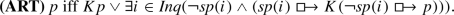This is a bit of a mouthful, so let me spell it out: p is actually true if and only if either (i) p is actually known (at some time), or (ii) it is metaphysically possible to know that actually p, which means that there is a course of inquiry i that is not actually successfully pursued, but if successfully pursued would lead to the knowledge that hadn’t it been pursued, p. Roughly, (ART) arises from ($$\alpha$$K’) by specifying $$\alpha$$ to be $$\lnot \textit{sp}(i)$$, and replacing $$\Diamond$$ by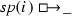for some suitable i.

(ART) can be understood as a claim about how extensive our means to obtain knowledge are. The anti-realist claims that these means are as extensive as truth itself. A realist, then, may claim that for some truths, there are no means that impart (even counterfactual) knowledge about them. However, the realist must now produce a more substantial case than appealing to the Fitch proposition that p and p is not known.

As seen in the example (Comet), there is an intuitive sense in which Fitch propositions are knowable. (ART) makes this sense precise. If $$p \wedge \lnot Kp$$ and (ART) is true, there is a course of inquiry i and a possible world w such that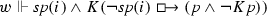(a world in which, say, an experiment succeeded that actually did not succeed). Nothing paradoxical follows from this, since from the successful pursuit of i or from the knowledge of the counterfactual it does not follow that $$w\Vdash K(p\wedge \lnot Kp)$$.

One may wonder what kind of i is suitable to witnessing the knowability of a truth p. I cannot give a general recipe. For many p, a suitable i is a procedure that is known to determine whether p without changing the truth value of p. If one pursues such an i for a true p, then one can counterfactually know that (even) if i hadn’t been pursued, p would (still) be true precisely because pursuing i imparted the knowledge that p, and one moreover knows that pursuing i did not change the truth of p.

However, this is not generally the case. Sometimes, pursuing some i imparts the knowledge that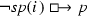without imparting the knowledge that p. The most salient example in this discussion are Fitch propositions $$p = (q \wedge \lnot Kq)$$: one can know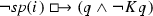, but one cannot come to this knowledge by learning that $$q \wedge \lnot Kq$$, since it is paradoxical to know this. But this does not conflict with (ART). As in (Comet), the knowability of a Fitch proposition $$q \wedge \lnot Kq$$ can be witnessed by a course of inquiry i that imparts the knowledge that q without changing the truth value of q, plus the background knowledge that pursuing i is the only way to know q. Then, if one successfully pursues i, one can learn that even if i hadn’t been pursued, q would obtain and moreover that q would not be known (since i is the only way to learn q).

There are also K-free examples of propositions p where one can come to know thatwithout knowing that p. One salient class are propositions denoting properties susceptible to an observer effect, i.e. a property that changes when one measures it. An example is (Hydrogen).

(Hydrogen).:

Suppose I have a container that contains either helium or hydrogen. It is actually true that p = the container contains hydrogen, but this is not known. Further suppose that any course of inquiry that could tell anyone anything about the container’s contents would involve opening it. In any possible world where someone does so, the gas escapes, leaving the container empty.

In (Hydrogen), any world where I make an attempt to determine whether p is a world in which p is false (since the gas escaped). So in these worlds p is not known. In worlds where p is true, it is not known that p, since any inquiry that does not involve opening the container fails to impart knowledge about p. So there is no course of inquiry i that imparts the knowledge that p. Formally,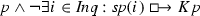.

Observer effects are counterexamples to the verificationist thesis that for every truth, there is a course of inquiry that verifies it. However, the failure of the verificationist thesis is compatible with the anti-realist thesis (ART), which only requires that if p is actually true, then there is some $$i \in \textit{Inq}$$ such that for the closest world w where $$\textit{sp}(i)$$,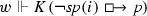. It is not required that p is known at w or even that p is true at w. For (Hydrogen), such an i would be to open the container and hold a flame to the escaping gas. Say that in the closest world where I do so, the gas will light, which imparts the knowledge that it is hydrogen (and not helium). Hence, in this world I know the counterfactual that had I not opened the container, it would contain hydrogen. I do not, however, know that the container contains hydrogen, since this proposition is false at that world.

There is a host of other cases that are similar to (Hydrogen), e.g. measuring voltage (adding a voltmeter to a conductor changes the resistance, hence changes voltage) or measuring air pressure in a tire (some air must escape into the measuring apparatus, changing the pressure of the tire). None of them are in obvious conflict with (ART).Footnote 6 Suitable inquiries for these cases might involve, say, mathematically correcting for the distortion introduced by measurement.

In any case, the claim that (ART) is true means that even for propositions p susceptible to observer effects, there are courses of inquiry that allow one to determine that p was the case before the inquiry was pursued, even if the inquiry necessarily involves changing the state. A suitable inquiry needs to be determined on a case by case basis—(ART) merely claims that there is one. My goal here is not to defend the truth of (ART), but only the coherence of (ART) vis-á-vis the gamut of triviality objections.

### How (ART) avoids the triviality objections

First, one can demonstrate that the right-hand-side of (ART) expresses a factive notion of knowability. Note the following plausible assumption about the semantics of counterfactual conditionals. For arbitrary formulae $$\varphi$$ and arbitrary worlds x and y with $$x \Vdash \varphi$$ and $$y \Vdash \lnot \varphi$$: if x is the closest $$\varphi$$-world to y, then y is the closest $$\lnot \varphi$$-world to x. Call this property Symmetry. Now, recall (ART).Take a proposition p for which the right-hand side of (ART) is true. If Kp then p is actually true. Otherwise, there is an i such that for the $$\textit{sp}(i)$$-world w that is closest to the actual world,. Since knowledge is factive,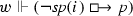. By Symmetry, the closest $$\lnot \textit{sp}(i)$$-world to w is the actual world. Thus, p is true in the actual world. Hence, knowing in the closest $$\textit{sp}(i)$$-world thatis knowing something about the actual world. In particular, no falsities are knowable.

That is, like Edgington (1985), I propose that someone in a non-actual world (situation) can know that actually p by having counterfactual knowledge about a description $$\alpha$$. But I make two clarifications: $$\alpha$$ is now clearly defined to be $$\lnot \textit{sp}(i)$$ for some i that depends on p; and knowledge about $$\alpha$$ does not constitute knowledge of actuality in every world (but only in the closest $$\textit{sp}(i)$$-world).

Hence, knowledge about $$\alpha =\lnot \textit{sp}(i)$$ is non-trivial, because in worlds that are not $$\textit{sp}(i)$$-closest to the actual world, knowledge about $$\alpha$$ is not knowledge of actuality. In particular,might fail at those worlds. Hence it is not the case that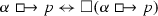and so (ART) avoids the simple triviality argument.

Similarly, (ART) also avoids the Williamsonian version of the triviality objection (which was a worry that Edgington (2010) left open). Let p be a contingent actually true proposition whose knowability is witnessed by some course of inquiry i. We can assume that $$\lnot p$$ and $$\lnot \textit{sp}(i)$$ are compossible (but see below). Let w be a witness for $$\Diamond (\lnot p \wedge \lnot \textit{sp}(i))$$. Since w satisfies neither p nor $$\textit{sp}(i)$$, it is false at w that. But of course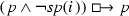is a logical truth and hence true at w. Thus, the following biconditional is false at w: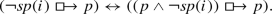But this means that this biconditional is not necessary. Similarly, it is not in conflict with (ART) to suppose that the biconditional is not necessarily known: since its right-hand-side is a logical truth, the biconditional is known wheneverknown. Butmay be false at some worlds, so to know it is non-trivial.

Of course, in the world that witnesses the knowability of p (i.e. the $$\textit{sp}(i)$$-world closest to the actual world),is true and known, and so the biconditional is true and known. But the contingent truth or knowledge of a biconditional does not mean that knowing its one side is equally good as knowing its other side. For comparison, consider that it happens to be the case in the actual world that grass is green iff $$1=1$$, since both sides of that biconditional are true. But the fact that knowledge of grass is green is substantive knowledge about the actual world is of course not incompatible with knowledge of $$1=1$$ being trivial knowledge. This is because grass is green iff $$1=1$$ is neither necessary nor trivial to know.

Similarly, knowing a contingently true counterfactual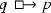is substantive knowledge about some possibly non-actual world, despite the biconditionaliff $$1=1$$ being (contingently) true.Footnote 7 In perfect analogy, there are worlds where knowing thatis substantive knowledge about actuality, even though in these worlds the counterfactual is contingently equivalent to the triviality that.

The assumption that $$\lnot p$$ and $$\lnot \textit{sp}(i)$$ are compossible hides a final difficulty, and pressing this difficulty seems to reveal another kind of triviality. Take any $$i\in \textit{Inq}$$ and let $$p = \lnot \textit{sp}(i)$$. Obviously, $$\lnot p$$ and $$\lnot \textit{sp}(i)$$ are not compossible. So the above biconditional is necessary for these i and p. Then, indeed, it is trivial that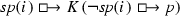because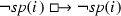is a logical truth. So (ART) trivialises knowability of which courses of inquiry are actually pursued. But this does not seem to be a trivial kind of knowledge. More generally, (ART) trivialises the knowability of all actual truths that logically follow from not having performed some course of inquiry.

But these cases are a distraction. The anti-realist can simply rewrite (ART) to demand that the pursuit of the i that witnesses the knowability of a proposition p be independent of p. As said above, (ART) should be read as a thesis about how extensive our means to gain knowledge are. To say that pursuing these means ought to be independent of the proposition whose knowability they witness simply amounts to a slightly stronger thesis. To do so formally, one can revise (ART) to (ART’): (I have underlined the added clause for visibility):

For those propositions p that necessarily are known to follow from $$\lnot \textit{sp}(i)$$ for some $$i \in \textit{Inq}$$, the refined principle (ART’) demands that p’s knowability be witnessed by another $$j \in \textit{Inq}$$. This demand rules out trivialising knowledge about which i are pursued: to know that some i is not pursued, one needs to pursue some j that is not i.

There is a formal point here about necessary propositions p that are never actually known (say, that some outrageously large number is prime, cf. Tennant 1997). To say that such a p is knowable should be to say that there is some i (say, a process of factorisation) such that. But this does not follow from (ART’), since if p is a logical truth, then $$\Box K(\lnot \textit{sp}(i)\rightarrow p)$$ is vacuously true for any i. However, this point is moot as long as we are operating within the idealised modal framework where knowledge is closed under inference (hence where all logical truths are known). In the present framework, logical truths are not counterexamples to (ART’), since if p is a logical truth, Kp is true, so the right-hand-side of (ART’) is true. If we move to a framework where not all logical truths are known, then $$\Box K(\lnot \textit{sp}(i)\rightarrow p)$$ can fail for some i, and (ART’) entails—as it should—that for some such i,I should note that, despite appearances, moving from (ART) to (ART’) does not put me in the idle business of chasing exceptions and adding stop-gap fixes. Rather, I am still offering increasingly precise formalisations of a single claim. That claim is now recognisable to be (*).

All examples and arguments I have presented so far, including those that motivated (AK), also motivate and defend (*). Aside from (Oats) and (Comet), mundane examples for (*) are abound, e.g. the procedure i to look at the ceiling which, if successfully pursued, would impart the knowledge that hadn’t I looked, there (still) would be a fly on the ceiling (Edgington 1985). An anti-realist following me on characterising knowability as in (ART) claims that such i’s can be found for all actual truths p (which would mean that all actual truths are knowable, in my sense of knowability).

In (ART) I have formalised the ‘would impart the knowledge’ of (*) simply as a counterfactual conditional. As seen, this trivialises the knowability of which inquiries are pursued. I find that (*) loses no plausibility when I spell out ‘would impart the knowledge’ to include that the successful pursuit of i must be relevant to the knowledge that hadn’t i been successfully pursued, p. All the same examples still apply and, in fact, the move to demand relevance only seems to sharpen and clarify what is meant by ‘impart’ in (*).

To give proper due to this, one could introduce the concept of a counterfactual relevant conditional and use it to phrase (ART). But sticking to the usual counterfactual semantics, one can approximate such a conditional by moving from (ART) to (ART’). Observe that whenever $$\lnot \textit{sp}(i)\rightarrow p$$ is necessarily known, then successfully pursuing i is not relevant to the knowledge that. These are exactly the cases that are excluded by the added clause in (ART’). So (ART’) derives from (ART) not as a stop-gap measure, but as a more precise way to spell out (*).

Finally, an anonymous reviewer points out to me another kind of triviality argument that has recently been developed by Heylen (2018). He argues that even if Edgington’s principle (AK), repeated below, is interpreted in a suitable semantics for actuality that avoids the derivations of every truth is known, it still follows that possibly, every truth is known which is equally unwelcome (or so Heylen argues).Footnote 8 The argument goes as follows.

(AK) $$Ap \rightarrow \Diamond KAp$$.

To avoid the simple triviality argument from $$Ap \leftrightarrow \Box Ap$$, Heylen follows Rabinowicz and Segerberg (1994) in interpreting Edgington’s principle (AK) in a semantics where worlds are indexed with different reference worlds. One evaluates propositions at a world as usual, except that Ap is true at a world iff p is true at that world’s reference world. Since what is the reference world may vary across epistemic accessibility, it does not follow from p being true at the actual reference world that Ap is true at all epistemically accessible worlds. Hence Ap does not trivially entail KAp.

However, Heylen (2018, Theorem 2.1) points out, the frame condition for (AK) on such semantics is this: given a reference world v and any metaphysically accessible w, all from w epistemically accessible worlds must have the reference world v. Then, suppose that the actual world is the reference world and that p is actually true. According to (AK) there is a w where KAp. That is, all worlds epistemically accessible to w satisfy Ap. All these worlds share the actual world as reference world by the frame condition. But this means that for any q that is actually true, Aq is true at all worlds epistemically accessible to w. Hence, all actual truths are known at w. So, possibly, every actual truth is known to be actually true.

This argument does not apply to (ART’), simply because (ART’) replaces the actuality operator A with a counterfactual that only corresponds to actuality in the $$\textit{sp}(i)$$-world closest to the actual world. To wit: If p is actually true, there is an i such that in the $$\textit{sp}(i)$$-world w closest to the actual world,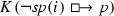is true. This means that all worlds epistemically accessible to w maketrue. But this does not mean that the actual world is the $$\lnot \textit{sp}(i)$$-world closest to these worlds. Consider that a v that is epistemically accessible from w may differ quite substantially from w (sharing only those truths that are known at w) and so be distant from w and hence also from the actual world (which is close to w). Therefore, it does not follow that for any other actually true q it is the case that all these worlds make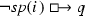true.

There is an entirely technical point here. Abstracting somewhat, Heylen makes a general objection to factive notions of knowability: for a knowability principle to be factive, its frame condition must be such that the worlds witnessing the knowability of some p are all accessing or referencing the actual world (lest actual falsities be knowable). But if this is the case, then these worlds witness the knowability of all actual p, entailing that possibly, all truths are known. Such an argument cannot work for (ART’), since (ART’) replaces modal operators (such as $$\Diamond$$ or A) with counterfactual conditionals. There are no frame conditions for counterfactuals, since—as opposed to $$\Diamond$$ and A—which world they access depends partly on valuation, not exclusively on the frame.

## Summary and conclusion

As is known since Edgington ’s landmark 1985 paper, Fitch’s paradox does not arise when the anti-realist principle is taken to be about counterfactual knowledge of actual truth. This reveals the paradox to be a misunderstanding: due to the imprecisions of casual talk about truth and actuality, truth is what is knowable has not been recognised for the claim that it should be: actual truth is what is possible to know to be actual.

But this explication of the anti-realist principle requires that there be non-trivial counterfactual knowledge of actual truth. Williamson’s (1987) forceful objection suggests that no such thing can exist. However, the solution (ART’) developed in the foregoing discussion shows that this is not so.

(ART’) can be considered a formalisation of the informal paraphrase (*), which itself is an explication of the knowability principle.

Williamson’s triviality objection is deflected by appealing to a restricted class of metaphysically possible worlds: those that diverge from the actual world in the counterfactual pursuit of some inquiry i. In such worlds, it is possible to have non-trivial counterfactual knowledge of the actual world by being acquainted with a description of the actual world, namely $$\alpha = \lnot \textit{sp}(i)$$. This explanation of counterfactual knowledge addresses Williamson’s general objection that counterfactual knowledge of actuality is philosophically unsound: it requires no more than there being true counterfactuals and there being knowledge of counterfactuals, which puts it on philosophically steady ground. On the technical side, Williamson’s argument is avoided by the observation that the trivialising biconditional is not a metaphysical necessity.

In conclusion, the anti-realist has at her disposal a version of the thesis that truth is what is knowable that is metaphysically sound and clarifies the relevant notions so that Fitch’s paradox disappears. But this just means that her thesis stands up to the Fitch objection—not that her thesis is true. I happen to think that it is not. However, the right-hand-side of (ART’) expresses a notion of knowability that is useful even if (ART’) itself is false. By appealing to the counterfactual pursuit of inquiries, we find a notion of knowability that is factive and on which mundane facts that intuitively seem knowable—such as there being N oats in my bowl—are in fact knowable. I leave open here whether all—and if not, how many—facts are knowable in this sense.

1. Some solutions to Fitch’s paradox discard the desideratum that knowability is factive. Notably, Fischer (2013) and Artemov and Protopopescu (2013) claim that only stable truths are knowable, which they express as $$\Box p \rightarrow \Diamond Kp$$, where the modal operators range over stages of verification. They argue that truths that become false in the process of verification are not knowable; conversely, then, falsities that become true at some stage count as knowable, falsifying factiveness. Since, arguably, the anti-realist position is characterised by an identification of truth and ideal verification (Kvanvig 1995), these accounts serve as clever defences of some epistemological thesis, but it is questionable how much they can aid the anti-realist.

2. Using only established concepts should not count as a decisive advantage, but it benefits the dialectic: the realist and anti-realist can have a sure-footed debate when the anti-realist’s thesis is stated in clear, well-understood and independently investigated terms. An instructive case is the debate between Williamson (2000, 2009) and Tennant (2001, 2002, 2010) that is arguably stifled by their disagreement about the logic and metaphysical properties of Tennant’s epistemic possibility modality.

3. This example is broadly similar to some examples used by Fara (2010). He uses such cases to defend that knowable derives from having certain capacities: I have the capacity to count, hence the capacity to know, even if I in fact did not count and hence won’t ever know.

4. The uniqueness of closest situations is convenient here, but nothing rests on it.

5. The idea to ground knowability in the possible pursuits of knowledge-inducing courses of inquiry has been anticipated by Artemov and Protopopescu (2013) and Fischer (2013). My proposal is different in that I consider a counterfactual conditional in the worlds that result from these possible pursuits. This allows me to retain factiveness, which Artemov and Protopopescu and Fischer must discard (see an earlier footnote). Their proposals also only make some truths knowable (those they call stable truths), whereas my proposal allows for the knowability of all actual truths, including Fitch propositions.

6. Note that uncertainty principles in quantum mechanics are not observer effects, but describe fundamental properties of quantum systems. It is true that Heisenberg’s principle, if correct, entails that one cannot know, not even counterfactually, that this particle has position x and momentum y for x and y below the threshold of possible precision of measurement. But this may just mean that there is no true proposition of the form this particle has position x and momentum y. See Putnam (1981, 1982) for discussion.

7. Some may argue that knowledge ofis not knowledge about some other world, but knowledge about the causal structure of the actual world. Those may consider my talk of possible worlds as mere convenience, and interpret (ART) as a statement about the causal structure of the actual world.

8. Artemov and Protopopescu (2013) point out that their stable knowability principle entails possible omniscience. They do not see this as a problem, but as “entirely what one would expect from the idea that all truths are knowable” (p. 3370). I will not adjudicate.

## References

• Artemov, S., & Protopopescu, T. (2013). Discovering knowability: A semantic analysis. Synthese, 190(16), 3349–3376.

• Beall, J. C. (2000). Fitch’s proof, verificationism, and the knower paradox. Australasian Journal of Philosophy, 78(2), 241–247.

• Brogaard, B., & Salerno, J. (2013). Fitch’s paradox of knowability. In E. N. Zalta (Ed.), The Stanford encyclopedia of philosophy, winter (2013th ed.). Stanford: Metaphysics Research Lab, Stanford University.

• Dummett, M. A. (1991). The logical basis of metaphysics. Cambridge: Harvard University Press.

• Edgington, D. (1985). The paradox of knowability. Mind, 94(376), 557–568.

• Edgington, D. (2010). Possible knowledge of unknown truth. Synthese, 173(1), 41–52.

• Fara, M. (2010). Knowability and the capacity to know. Synthese, 173(1), 53–73.

• Fischer, M. (2013). Some remarks on restricting the knowability principle. Synthese, 190(1), 63–88.

• Fuhrmann, A. (2014). Knowability as potential knowledge. Synthese, 191(7), 1627–1648.

• Heylen, J. (2018). Factive knowability and the problem of possible omniscience. Philosophical Studies. https://doi.org/10.1007/s11098-018-1180-x.

• Kvanvig, J. (1995). The knowability paradox and the prospects for anti-realism. Noûs, 29(4), 481–500.

• Putnam, H. (1981). Quantum mechanics and the observer. Erkenntnis, 16(2), 193–219.

• Putnam, H. (1982). Why there isn’t a ready-made world. Synthese, 51(2), 141–167.

• Rabinowicz, W., & Segerberg, K. (1994). Actual truth, possible knowledge. Topoi, 13(2), 101–115.

• Rückert, H. (2004). A solution to fitch’paradox of knowability. In S. Rahman & J. Symons (Eds.), Logic, epistemology, and the unity of science (pp. 351–380). Berlin: Springer.

• Tennant, N. (1997). The taming of the true. Oxford: Oxford University Press.

• Tennant, N. (2001). Is every truth knowable? Reply to Williamson. Ratio, 14(3), 263–280.

• Tennant, N. (2002). Victor vanquished. Analysis, 62(2), 135–142.

• Tennant, N. (2009). Revamping the restriction strategy. In J. Salerno (Ed.), All truths are known: New essays on the knowability paradox. Oxford: Oxford University Press.

• Tennant, N. (2010). Williamsons woes. Synthese, 173(1), 9–23.

• Williamson, T. (1987). On the paradox of knowability. Mind, 96(382), 256–261.

• Williamson, T. (2000). Tennant on knowable truth. Ratio, 13(2), 99–114.

• Williamson, T. (2009). Tennant’s troubles. In J. Salerno (Ed.), Every truth is known: New essays on knowability (pp. 183–204). Oxford: Oxford University Press.

## Author information

Authors

### Corresponding author

Correspondence to Julian J. Schlöder.